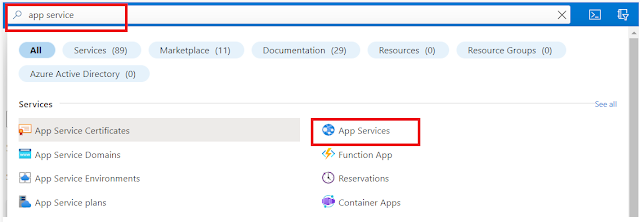### Image Background Removal using Python

In the world of image processing and manipulation, one common task is removing backgrounds from images. This can be particularly useful for creating visually appealing graphics or preparing images for various applications. One tool that helps achieve this is REMBG, a Python library designed for background removal from images. In this blog post, we'll guide you through a simple Python program that utilizes REMBG to remove backgrounds from images

Setting Up the Environment:

Before we dive into the code, let's ensure we have the necessary Python libraries installed:

1. rembg: A Python library that will handle the background removal for us.

``pip install rembg``
2. PIL (Python Imaging Library): A Python library for image processing.

``pip install pillow``
3. easygui: A GUI library for file selection.

``pip install easygui``

### LeetCode Solution - Problem Valid Parentheses

• Given a string s containing just the characters '(', ')', '{', '}', '[' and ']', determine if the input string is valid.
• An input string is valid if:
• Open brackets must be closed by the same type of brackets.
• Open brackets must be closed in the correct order.
• Every close bracket has a corresponding open bracket of the same type.
Example 1:

``````Input: s = "()"
Output: true
``````

### LeetCode Solution - Problem Longest Substring Without Repeating Characters

• Given a string s, find the length of the longest substring without repeating characters.
Example 1:

``````Input: s = "abcabcbb"
Output: 3
Explanation: The answer is "abc", with the length of 3.``````

### LeetCode Solution - Problem Repeated DNA Sequences

• The DNA sequence is composed of a series of nucleotides abbreviated as 'A', 'C', 'G', and 'T'.
• For example, "ACGAATTCCG" is a DNA sequence.
• When studying DNA, it is useful to identify repeated sequences within the DNA.
• Given a string s that represents a DNA sequence, return all the 10-letter-long sequences (substrings) that occur more than once in a DNA molecule. You may return the answer in any order.
Example 1:

``````Input: s = "AAAAACCCCCAAAAACCCCCCAAAAAGGGTTT"
Output: ["AAAAACCCCC","CCCCCAAAAA"]
``````

### LeetCode Solution - Problem Longest Common Prefix

• Write a function to find the longest common prefix string amongst an array of strings.
• If there is no common prefix, return an empty string "".
Example 1:

``````Input: strs = ["flower","flow","flight"]
Output: "fl"
``````

### LeetCode Solution - Problem Reverse String

• Write a function that reverses a string. The input string is given as an array of characters s.
• You must do this by modifying the input array in-place with O(1) extra memory.
Example 1:

``````Input: s = ["h","e","l","l","o"]
Output: ["o","l","l","e","h"]
``````

### LeetCode Solution - Problem Pow(x, n)

• Implement pow(x, n), which calculates x raised to the power n (i.e., x^n).
Example 1:

``````Input: x = 2.00000, n = 10
Output: 1024.00000
``````

### LeetCode Solution - Problem Factorial Trailing Zeroes

• Given an integer n, return the number of trailing zeroes in n!.
• Note that n! = n * (n - 1) * (n - 2) * ... * 3 * 2 * 1.
Example 1:

``````Input: n = 3
Output: 0
Explanation: 3! = 6, no trailing zero.
``````

### LeetCode Solution - Problem Palindrome Number

• Given an integer x, return true if x is a palindrome, and false otherwise.
Example 1:

``````Input: x = 121
Output: true
Explanation: 121 reads as 121 from left to right and from right to left.
``````

### LeetCode Solution - Problem Reverse Integer

• Given a signed 32-bit integer x, return x with its digits reversed. If reversing x causes the value to go outside the signed 32-bit integer range [-231, 231 - 1], then return 0.
• Assume the environment does not allow you to store 64-bit integers (signed or unsigned).
Example 1:

``````Input: x = 123
Output: 321
``````

### LeetCode Solution - Problem Single Number

• Given a non-empty array of integers nums, every element appears twice except for one. Find that single one.
• You must implement a solution with a linear runtime complexity and use only constant extra space.
Example 1:

``````Input: nums = [2,2,1]
Output: 1
``````

## You might also like

### Deploy your Django web app to Azure Web App using App Service - F1 free plan

In this post, we will look at how we can deploy our Django app using the Microsoft Azure app service - a free plan. You need an Azure accoun...